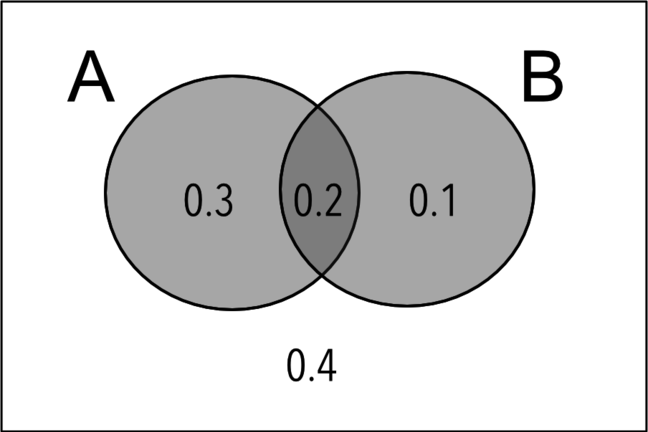## Want to keep learning?

This content is taken from the Cambridge University Press's online course, Teaching Probability. Join the course to learn more.
3.5

## Cambridge University PressVenn diagrams can be used to solve a range of problems

# Probability, sets, and Venn diagrams

In this step, we review a further selection of related topics in probability, and provide sample examination-style questions for you to try.

This step covers:

• Using complements

• Mutually exclusive and exhaustive events

• Using sets and Venn diagrams

We address each of these in turn. After the description of the topics you will find the sample questions, which can be answered on paper.

You can reflect on this material and share your answers in the comments; our solutions, and a chance to discuss classroom approaches, are provided in the next step.

### 1. Using complements

This is simply the idea that if the probability of an event happening is P, then the probability that it does not happen is 1 – P.

### 2. Mutually exclusive and exhaustive events

Events are mutually exclusive if they cannot occur simultaneously.

• In many of the practical situations that we have encountered in this course, this condition arises quite naturally. For example, when using a spinner, the outcome may be red or green (say), but it could not be both.

• As an example of a pair of events that are not mutually exclusive, suppose we roll an ordinary dice and consider the events ‘the score is even’ and the ‘score is 4’. Since these two results clearly could happen together, they are not mutually exclusive.

Events are exhaustive if between them they cover ‘all the possibilities’.

• The probabilities of a set of mutually exclusive and exhaustive events will always add to 1.

### 3. Using sets and Venn diagrams

As we saw in previous weeks, the language of sets is a natural way to describe combinations of events.

• We can use unions of sets where we want to express the idea that one event or another happens; the entire shaded region in the Venn diagram above represents the probability of event A or event B occurring; in set notation this would be written as p(A⋃B), and its value in the example above is 0.6.

• Similarly, intersections of sets occur when one event and another occur. the overlapping section at the centre of the Venn diagram above shows the probability of event A and B occurring, or p(A⋂B) in set notation (equal to 0.2 in this example).

Venn diagrams typically show two or three overlapping sets, and like tree diagrams they can be used to show probabilities or frequencies.

## Sample questions

### Question 1

If you throw a particular drawing-pin in the air, the probability of it landing with its point up is 0.35. What is the probability of it coming down without its point up?

### Question 2

A tube of small chocolate sweets has four different colours.

• There are twice as many blue sweets as red sweets.

• There are three times as many green sweets as blue sweets.

• There are also some brown sweets.

The probability of choosing a red sweet is 0.05. What is the probability of picking a brown sweet?

### Question 3

40 students are asked whether they watch football, tennis or rugby on TV.

• 10 say they don’t watch any sport on TV.

• 5 watch all three sports.

• 15 watch football and tennis, but do not watch rugby.

• 6 watch football and rugby.

• 24 watch football.

• 7 watch tennis and rugby.

• 4 watch only tennis.

a Represent this information on a Venn diagram.

b If a student is picked at random, what is the probability that they watch tennis?